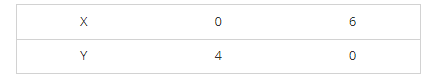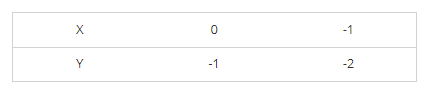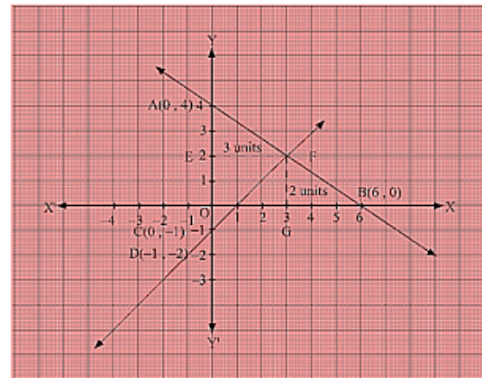# Draw the graphs of the following linear equations on the same graph paper:Question:

Draw the graphs of the following linear equations on the same graph paper: 2x + 3y = 12, x - y = 1 Find the coordinates of the vertices of the triangle formed by the two straight lines and the y-axis. Also, find the area of the triangle.

Solution:

We are given, 2x +3y =12

We get,

$y=\frac{12-2 x}{3}$

Now, substituting x = 0 in

$y=\frac{12-2 x}{3}$

we get y = 4

Substituting x = 6 in

$y=\frac{12-2 x}{3}$

we get y = 0

Thus, we have the following table exhibiting the abscissa and ordinates of points on the line represented by the given equationPlotting A (0, 4) and E(6, 0) on the graph and by joining the points ,

we obtain the graph of equation 2x + 3y = 12.

We are given, x – y = 1

We get, y = x – 1

Now, substituting x = 0 in y = x - 1, we get y = -1

Substituting x in y = x - 1, we get y = -2

Thus, we have the following table exhibiting the abscissa and ordinates of points on the line represented by the given equationPlotting D (0, – 1) and E (-1, 0) on the graph and by joining the points, we obtain the graph of equation x - y = 1.By the intersection of lines formed by 2x + 3y = 12 and x - y = 1 on the graph, triangle ABC is formed on y axis.

Therefore, AC at y axis is the base of triangle ABC having AC = 5 units on y axis.

Draw FE perpendicular from F on y axis.

FE parallel to x axis is the height of triangle ABC having FE = 3 units on x axis.

Therefore, Area of triangle ABC, say A is given by A = (Base × Height)/2 = (AC × FE)/2 = (5× 3)/2 =>15/2 = 7.5 sq. units